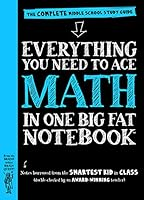# Everything You Need to Ace Math in One Big Fat Notebook: The Complete Middle School Study Guide## Book Description

It’s the revolutionary math just for middle school students from the brains behind Brain Quest.

Everything You Need to Ace Math . . . covers everything to get a student over any math hump: fractions, decimals, and how to multiply and divide them; ratios, proportions, and percentages; geometry; and probability; expressions and equations; and the coordinate plane and .

The BIG FAT NOTEBOOK™ series is built on a simple and irresistible conceit—borrowing the notes from the smartest kid in class. There are five books in all, and each is the only book you need for each main subject taught in middle school: Math, Science, American History, English Language Arts, and World History. Inside the reader will find every subject’s key concepts, easily digested and summarized: Critical ideas highlighted in neon . Definitions explained. Doodles that illuminate tricky concepts in marker. Mnemonics for memorable shortcuts. And quizzes to recap it all.

The BIG FAT NOTEBOOKS meet Common Core State Standards, Next Generation Science Standards, and state history standards, and are vetted by National and State Teacher of the Year Award–winning teachers. They make learning fun and are the perfect next step for every kid who grew up on Brain Quest.

UNIT 1: The Number System
Chapter 1: Types of Numbers and the Number Line
Chapter 2: Positive and Negative Numbers
Chapter 3: Absolute Value
Chapter 4: Factors and Greatest Common Factor
Chapter 5: Multiples and Least Common Multiple
Chapter 6: Fraction Basics: Types of Fractions and Adding and Subtracting Fractions
Chapter 7: Multiplying and Dividing Fractions
Chapter 8: Adding and Subtracting Decimals
Chapter 9: Multiplying Decimals
Chapter 10: Dividing Decimals
Chapter 11: Adding Positive and Negative Numbers
Chapter 12: Subtracting Positive and Negative Numbers
Chapter 13: Multiplying and Dividing Positive and Negative Numbers
Chapter 14: Inequalities

UNIT 2: Ratios, Proportions, and Percents
Chapter 15: Ratios
Chapter 16: Unit Rate and Unit Price
Chapter 17: Proportions
Chapter 18: Converting Measurements
Chapter 19: Percent
Chapter 20: Percent Word Problems
Chapter 21: Taxes and Fees
Chapter 22: Discounts and Markups
Chapter 23: Gratuity and Commission
Chapter 24: Simple Interest
Chapter 25: Percent Rate of Change
Chapter 26: Tables and Ratios

UNIT 3: Expressions and Equations
Chapter 27: Expressions
Chapter 28: Properties
Chapter 29: Like Terms
Chapter 30: Exponents
Chapter 31: Order of Operations
Chapter 32: Notation
Chapter 33: Square and Cube Roots
Chapter 34: Comparing Irrational Numbers
Chapter 35: Equations
Chapter 36: Solving for Variables
Chapter 37: Solving Multistep Equations
Chapter 38: Solving and Graphic Inequalities
Chapter 39: Word Problems with Equations and Inequalities

UNIT 4: Geometry
Chapter 40: Introduction to Geometry
Chapter 41: Angles
Chapter 43: Triangles and Area
Chapter 44: The Pythagorean Theorem
Chapter 45: Circles, Circumference, and Area
Chapter 46: Three-Dimensional Figures
Chapter 47: Volume
Chapter 48: Surface Area
Chapter 49: Angles, Triangles, and Transversal Lines
Chapter 50: Similar Figures and Scale Drawings

UNIT 5: Statistics and Probability
Chapter 51: Introduction to Statistics
Chapter 52: Measures of Central Tendency and Variation
Chapter 53: Displaying Data
Chapter 54: Probability

UNIT 6: The Coordinate Plane and Functions
Chapter 55: The Coordinate Plane
Chapter 56: Relations, Lines, and Functions
Chapter 57: Slope
Chapter 58: Linear Equations and Functions
Chapter 59: Simultaneous Linear Equations and Functions
Chapter 60: Nonlinear Functions
Chapter 61: Polygons and the Coordinate Plane
Chapter 62: Transformations
Chapter 63: Proportional Relationships and

## Book Details

• Title: Everything You Need to Ace Math in One Big Fat Notebook: The Complete Middle School Study Guide
• Author: ,
• Length: 528 pages
• Edition: Study Guide
• Language: English
• Publisher:
• Publication Date: 2016-08-09
• ISBN-10: 0761160965
• ISBN-13: 9780761160960